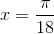## Example Questions

### Example Question #70 : Trigonometry

If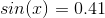, what is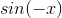?  Round to the nearest hundredth.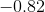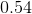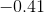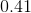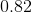Explanation:

Recall that the sine wave is symmetrical with respect to the origin. Therefore, for any value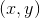, the value for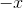is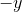. Therefore, if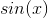is, then for, it will be.

### Example Question #71 : Trigonometry

In a right triangle, cos(A) =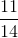. What is sin(A)?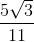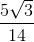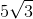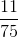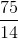Explanation:

In a right triangle, for sides a and b, with c being the hypotenuse,. Thus if cos(A) is, then c = 14, and the side adjacent to A is 11. Therefore, the side opposite of angle A is the square root of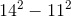, which is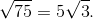Since sin is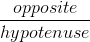, sin(A) is.

### Example Question #72 : Trigonometry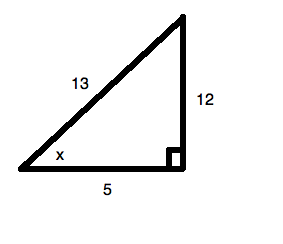What is the value of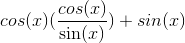?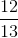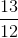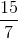Explanation:

As with all trigonometry problems, begin by considering how you could rearrange the question. They often have hidden easy ways out. So begin by noticing: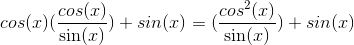Now, you can treatlike it is any standard denominator. Therefore: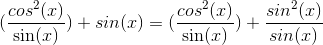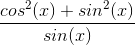Now, from our trig identities, we know that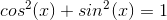, so we can say: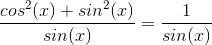Now, for our triangle, theis. Therefore,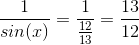### Example Question #73 : Trigonometry

Solve for: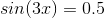if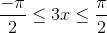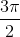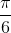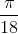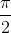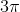Explanation:Recall that the standard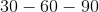triangle, in radians, looks like: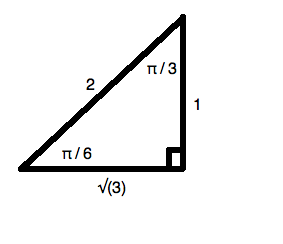Since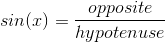, you can tell that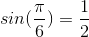.

Therefore, you can say thatmust equal: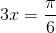Solving for, you get: